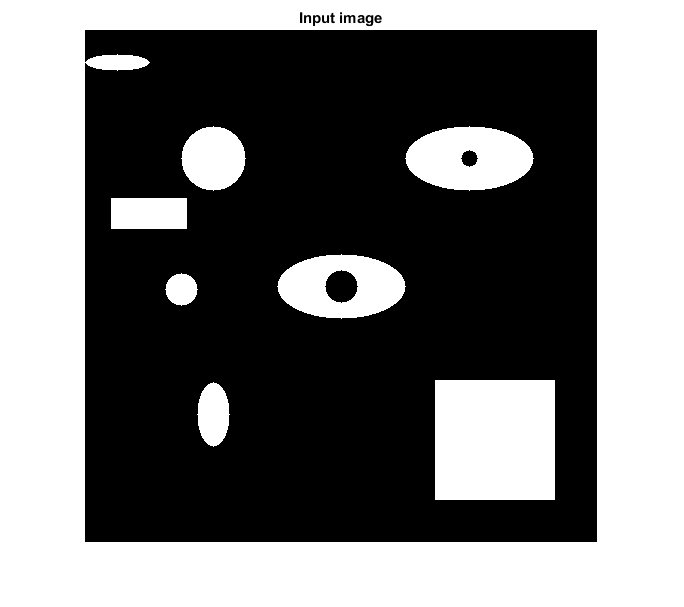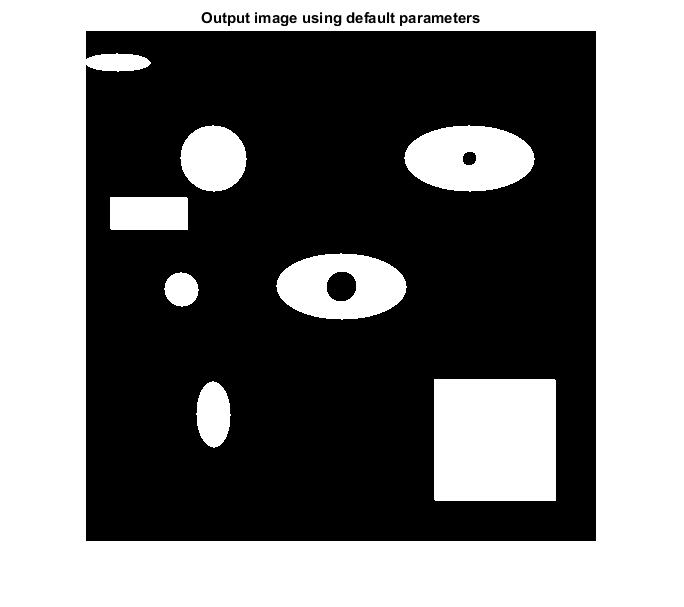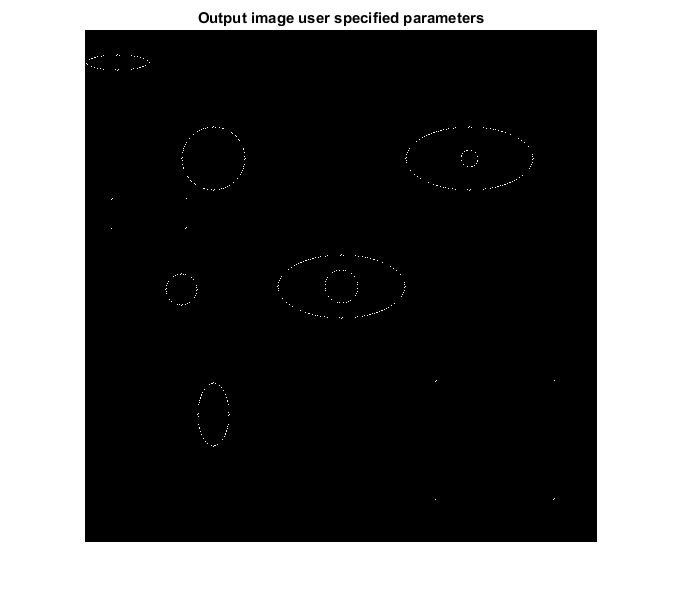# MORPHITERMOD_CVIP

morphitermod_cvip() - Iterative morphological modification of a binary image.

## SYNTAX

`outImage = morphitermod_cvip(binImage, setsSurr, n, boolFunc, rotate)`

Input Parameters include :

• binImage - Binary image of MxN size.
• setsSurr - A row vector containing the ids of the surrounds. The maximum length is 14. For examples, [2 5 7] or [1:14].
```                 surrounds id = 1  ---->  0 0 x
0 b 0
x 0 0```
```                 surrounds id = 2  ---->  0 1 x
0 b 0
x 0 0```
```                 surrounds id = 3  ---->  1 1 x
0 b 0
x 0 0
.
.
.```
```                 surrounds id = 14 ---->  1 1 x
0 b 0
x 1 1```
```                 If multiple surrounds are selected, a_ij = 1 if any of
the surrounds are present. See reference 1 for other
surrounds, which are not shown above.```
`                                                            (default: 7)`
• n - Number of iterations. (default: 1)
• boolFunc - Boolean function L(a,b)
`                 boolFunc = 1  ---->  !a     (i.e. NOT a)`
`                 boolFunc = 2  ---->  ab     (i.e a AND b)`
`                 boolFunc = 3  ---->  a+b    (i.e a OR b)`
`                 boolFunc = 4  ---->  a^b    (i.e a XOR b)`
`                 boolFunc = 5  ---->  (!a)b  (i.e (NOT a) AND b)`
`                 boolFunc = 6  ---->  (a!b)  (i.e a AND (NOT b))`
`                                                            (default: 1)`
• rotate - Rotate each surrounds set. Checks each surrounds set in all six directions if rotate option is set ON.
```                 rotate = 0  ---->  OFF
rotate = 1  ---->  ON```
`                                                            (default: 0)`

Output Parameter include :

• outImage - Output image of iterative morphological modification. It is of Boolean class.

## DESCRIPTION

The function performs an iterative morphological modification of a binary image. To perform morphological modification,first,a_ij is found for each pixel;a_ij is 1 if (i,j)th pixel has the user specified surrounds or 0 otherwise. Then,morphological operation is performed between a_ij and b_ij,where b_ij is current pixel value(either 1 or 0). The user can specify the morphological operation by choosing one of the boolean function from the pre-defined list. A total of 14 surrounds sets are available. Each surrounds set has six surrounding pixels as we have considered NW/SE six connectivity. If rotate option is set ON, the surrounds are checked in all six directions. The morophological modification is repeated n times.

## REFERENCE

1. Scott E Umbaugh. DIGITAL IMAGE PROCESSING AND ANALYSIS: Applications with MATLAB and CVIPtools, 3rd Edition.

## EXAMPLE

```% Read image

% Call function using default parameters

O1 = morphitermod_cvip(I);

% Number of iterations

n = 3;

% boolean function L(a,b) = ab

f = 2;

% sets of surrounds

S = [1 2 3 5];

% rotate OFF

r = 1;

% Call function using user specified parameters

O2 = morphitermod_cvip(I,S,n,f,r);

% Display input image

figure;imshow(I);title('Input image');

% Display output image

figure;imshow(O1,[]);title('Output image using default parameters');

figure;imshow(O2,[]);title('Output image user specified parameters');
```## CREDITS

Author: Norsang Lama, June 2017